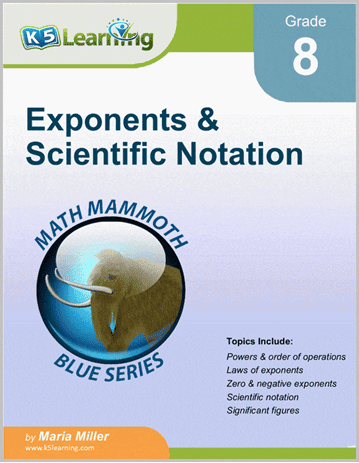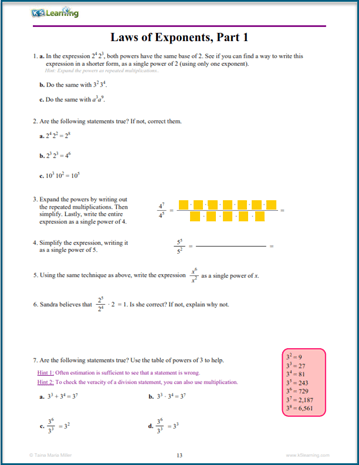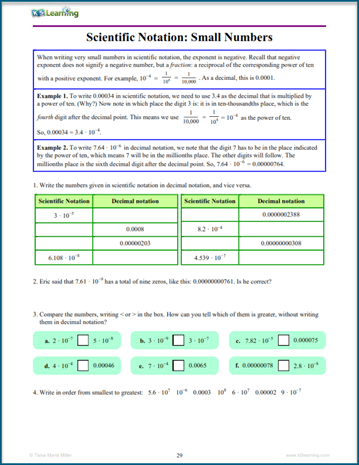# K5 Adds Exponents and Scientific Notation Workbook to its Bookstore

This is an advanced workbook for students ready to work on exponents and scientific notation. In the math curriculum, it’s a grade 8 level workbook.

## Exponents and scientific notation workbookThis math workbook on rational numbers is divided into 12 sections. Each section starts with a short tutorial with an example, followed by practice questions. The topics covered in this workbook are:

• powers & the order of operations,
• powers with negative bases,
• laws of exponents,
• zero and negative bases,
• scientific notation,
• significant digits.

## Sample exponents pages

If you’re curious what the pages look like, take a look at these sample pages: